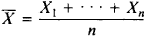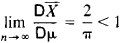# Estimator

(redirected from Is consistent for)
Also found in: Dictionary, Thesaurus, Medical.
Related to Is consistent for: Consistent estimator

## estimator

[′es·tə‚mād·ər]
(statistics)
A random variable or a function of it used to estimate population parameters.

## Estimator

in statistics, a function of the results of observations that is used to estimate an unknown parameter of the probability distribution of random variables that are under study. In English, a distinction is sometimes, but not always, made between the terms “estimator” and “estimate”: an estimate is the numerical value of the estimator for a particular sample.

Suppose, for example, that X1, . . . , Xn are independent random variables having the same normal distribution with the unknown mean a. Possible point estimators of a are the arithmetic mean of the observation resultsand the sample median μ = μ(X1,..., Xn).

In choosing an estimator of a parameter θ, it is natural to select a function θ*(X1, . . . , Xn) of the observation results X1, . . . , Xn that is in some sense close to the true value of the parameter. By adopting some measure of the closeness of an estimator to the parameter being estimated, different estimators can be compared with respect to quality. A commonly used measure of closeness is the magnitude of the mean squared error

Eθ(θ* – θ)2 = Dθθ* + (θ - Eθθ*)2

which is expressed here in terms of the mathematical expectation Eθθ* and variance Dθθ* of the estimator.

The estimator θ* is said to be unbiased if Eθθ* = θ. In the class of all unbiased estimators, the best estimators from the standpoint of mean squared error are those that have for a given n the minimum possible variance for all θ. The estimator X̄ defined above for the parameter a of a normal distribution is the best unbiased estimator, since the variance of any other unbiased estimator a* of a satisfies the inequality Daa* DaX̄ = σ2/n, where σ2 is the variance of the normal distribution. If a minimum-variance unbiased estimator exists, an unbiased best estimator can also be found in the class of functions that depend only on a sufficient statistic.

In constructing estimators for large n, it is natural to assume that as n → ∞, the probability of deviations of θ* from the true value of θ that exceed some given number will be close to θ. Estimators with this property are said to be consistent. Unbiased estimators whose variance approaches θ as n→ ∞ are consistent. Because the rate at which the limit is approached plays an important role here, an asymptotic comparison of two estimators is made by considering the ratio of their asymptotic variances. In the example given above, the arithmetic mean X̄ is the best, and consequently the asymptotically best, estimator for the parameter a, whereas the sample median μ, although an unbiased estimator, is not asymptotically best, sinceNonetheless, the use of μ sometimes has advantages. If, for example, the true distribution is not exactly normal, the variance of X̄ may increase sharply while the variance of μ remains almost the same—that is, μ has the property known as robustness.

A widely used general method of obtaining estimators is the method of moments. In this technique, a certain number of sample moments are equated to the corresponding moments of the theoretical distribution, which are functions of the unknown parameters, and the equations obtained are solved for these parameters. The method of moments is convenient to use, but the estimators produced by it are not in general asymptotically best estimators. From the theoretical point of view, the maximum likelihood method is more important. It yields estimators that, under certain general conditions, are asymptotically best. The method of least squares is a special case of the maximum likelihood method.

An important supplement to the use of estimators is provided by the estimation of confidence intervals.

### REFERENCES

Kendall, M., and A. Stuart. Statisticheskie vyvody i sviazi. Moscow, 1973. (Translated from English.)
Cramér, H. Matematicheskie metody statistiki, 2nd ed. Moscow, 1975. (Translated from English.)

A. V. PROKHOROV

## estimator

A person who, by experience and training, is capable of estimating the probable cost of a building or portion thereof.
Site: Follow: Share:
Open / Close